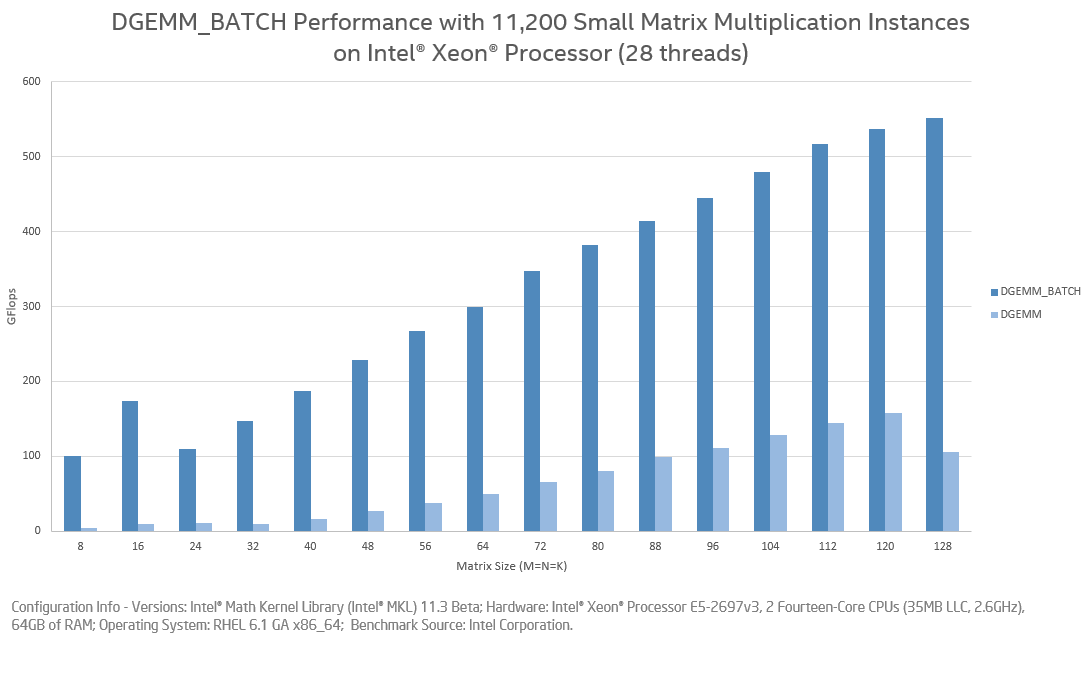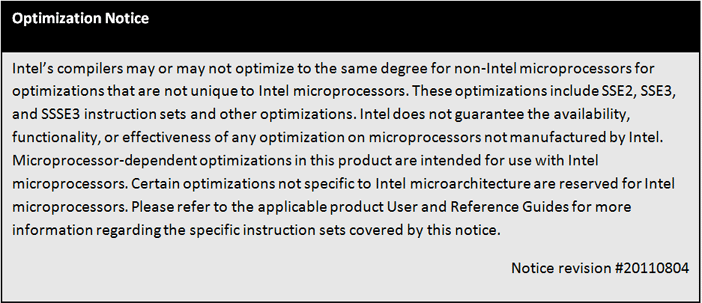# Introducing Batch GEMM Operations

ID 659731
Updated 4/9/2017
Version Latest
PublicBy

The general matrix-matrix multiplication (GEMM) is a fundamental operation in most scientific, engineering, and data applications. There is an everlasting desire to make this operation run faster. Optimized numerical libraries like Intel® oneAPI Math Kernel Library (oneMKL) typically offer parallel high-performing GEMM implementations to leverage the concurrent threads supported by modern multi-core architectures. This strategy works well when multiplying large matrices because all cores are used efficiently. When multiplying small matrices, however, individual GEMM calls may not optimally use all the cores. Developers wanting to improve utilization usually batch multiple independent small GEMM operations into a group and then spawn multiple threads for different GEMM instances within the group. While this is a classic example of an embarrassingly parallel approach, making it run optimally requires a significant programming effort that involves threads creation/termination, synchronization, and load balancing. That is, until now.

oneMKL 11.3 Beta (part of Intel® oneAPI Base & HPC Toolkit 2016 Beta) includes a new flavor of GEMM feature called "Batch GEMM". This allows users to achieve the same objective described above with minimal programming effort. Users can specify multiple independent GEMM operations, which can be of different matrix sizes and different parameters, through a single call to the "Batch GEMM" API. At runtime, oneMKL will intelligently execute all of the matrix multiplications so as to optimize overall performance. Here is an example that shows how "Batch GEMM" works:

#### Example

Let A0, A1 be two real double precision 4x4 matrices; Let B0, B1 be two real double precision 8x4 matrices. We'd like to perform these operations:

C0 = 1.0 * A0 * B0T  , and C1 = 1.0 * A1 * B1T

where C0 and C1 are two real double precision 4x8 result matrices.

Again, let X0, X1 be two real double precision 3x6 matrices; Let Y0, Y1 be another two real double precision 3x6 matrices. We'd like to perform these operations:

Z0 = 1.0 * X0 * Y0T + 2.0 * Z0, and Z1 = 1.0 * X1 * Y1T + 2.0 * Z1

where Z0 and Z1 are two real double precision 3x3 result matrices.

We could accomplished these multiplications using four individual calls to the standard DGEMM API. Instead, here we use a single "Batch GEMM" call for the same with potentially improved overall performance. We illustrate this using the "cblas_dgemm_batch" function as an example below.

#define    GRP_COUNT    2

MKL_INT    m[GRP_COUNT] = {4, 3};
MKL_INT    k[GRP_COUNT] = {4, 6};
MKL_INT    n[GRP_COUNT] = {8, 3};

MKL_INT    lda[GRP_COUNT] = {4, 6};
MKL_INT    ldb[GRP_COUNT] = {4, 6};
MKL_INT    ldc[GRP_COUNT] = {8, 3};

CBLAS_TRANSPOSE    transA[GRP_COUNT] = {'N', 'N'};
CBLAS_TRANSPOSE    transB[GRP_COUNT] = {'T', 'T'};

double    alpha[GRP_COUNT] = {1.0, 1.0};
double    beta[GRP_COUNT] = {0.0, 2.0};

MKL_INT    size_per_grp[GRP_COUNT] = {2, 2};

// Total number of multiplications: 4
double    *a_array, *b_array, *c_array;
a_array = A0, b_array = B0, c_array = C0;
a_array = A1, b_array = B1, c_array = C1;
a_array = X0, b_array = Y0, c_array = Z0;
a_array = X1, b_array = Y1, c_array = Z1;

// Call cblas_dgemm_batch
cblas_dgemm_batch (
CblasRowMajor,
transA,
transB,
m,
n,
k,
alpha,
a_array,
lda,
b_array,
ldb,
beta,
c_array,
ldc,
GRP_COUNT,
size_per_group);



The "Batch GEMM" interface resembles the GEMM interface. It is simply a matter of passing arguments as arrays of pointers to matrices and parameters, instead of as matrices and the parameters themselves. We see that it is possible to batch the multiplications of different shapes and parameters by packaging them into groups. Each group consists of multiplications of the same matrices shape (same m, n, and k) and the same parameters.

#### Performance

While this example does not show performance advantages of "Batch GEMM", when you have thousands of independent small matrix multiplications then the advantages of "Batch GEMM" become apparent. The chart below shows the performance of 11K small matrix multiplications with various sizes using "Batch GEMM" and the standard GEMM, respectively. The benchmark was run on a 28-core Intel Xeon processor (Haswell). The performance metric is Gflops, and higher bars mean higher performance or a faster solution.The second chart shows the same benchmark running on a 61-core Intel Xeon Phi co-processor (KNC). Because "Batch GEMM" is able to exploit parallelism using many concurrent multiple threads, its advantages are more evident on architectures with a larger core count.#### Summary

This article introduces the new API for batch computation of matrix-matrix multiplications. It is an ideal solution when many small independent matrix multiplications need to be performed. "Batch GEMM" supports all precision types (S/D/C/Z). It has Fortran 77 and Fortran 95 APIs, and also CBLAS bindings. It is available in oneMKL 11.3 Beta and later releases. Refer to the reference manual for additional documentation.